# Intro¶

This notebook takes us through the entire data science pipeline of importing and cleaning data, training and selecting models, and finally forming predictions on the unlabeled test data. These data represent the demographics and response/payment of a mail acquisition campain. The goal of this case is to use the 50k rows of labeled data to create a model that will give predictions to maximize revenue from 5,000 mailings sent to the top-scored recipients selected of the 50k-row unlabeled data set.

## Step 0: import libraries¶

In :
import pandas as pd
import numpy as np
import seaborn as sns
import matplotlib.pyplot as plt
from sklearn.ensemble import RandomForestClassifier, ExtraTreesClassifier, GradientBoostingClassifier
from sklearn.linear_model import LogisticRegression, LinearRegression
from sklearn.neighbors import KNeighborsClassifier
from sklearn.metrics import accuracy_score, confusion_matrix, roc_curve
from sklearn.pipeline import Pipeline
from sklearn.feature_selection import SelectFromModel
import time, math, pickle, itertools
import xgboost as xgb
%matplotlib inline

/home/luke/anaconda3/envs/tensorflow/lib/python3.5/site-packages/sklearn/cross_validation.py:44: DeprecationWarning: This module was deprecated in version 0.18 in favor of the model_selection module into which all the refactored classes and functions are moved. Also note that the interface of the new CV iterators are different from that of this module. This module will be removed in 0.20.
"This module will be removed in 0.20.", DeprecationWarning)


## Step 1: import and clean the data¶

In this step, we import the data from a CSV file, fill in missing values with the column mean values, create an id-to-payment dictionary, and encode all categorical data both in one-hot encoding and ordinal integers.

In :
# set random number generator seed
_seed = 0

# shuffle rows, index on W_ID, and remove trailing whitespace from column names
df = df.sample(frac=1, random_state=_seed).reset_index(drop=True)
df = df.set_index(df.W_ID)
df.columns = [c.strip() for c in df.columns.values]

# drop the column will all missing values
df = df.drop('colname',1)

# fill in missing values, replace missing continuous values with the average of the rows
df = df.replace(-1, np.nan)
cont_feats = df.select_dtypes(include=[np.number]).columns
df.loc[:,cont_feats] = df.loc[:,cont_feats].fillna(df.loc[:,cont_feats].mean())

# make a categorical variable of response amount
# df['payment_categorical'] = df['Payment'].astype('category')

# # properly process categorical variables
# for col in df.columns:
#     if df[col].dtype == 'object':
#         df.loc[:,col] = df.loc[:,col].astype('category')

# encode categories as dummy (ordinal and one-hot) variables and create scoring lookup dictionary
lookup_df = df[['W_ID', 'Payment']]
lookup_dict = df['Payment'].to_dict()
onehot_df = pd.get_dummies(df)
for feature in df.columns: # Loop through all columns in the dataframe
if df[feature].dtype.name == 'object': # Only apply for columns with categorical strings
df[feature] = pd.Categorical(df[feature]).codes # Replace strings with an integer


## Step 1.5: define some useful functions¶

We need to create some additional functionality that isn't in the libraries we will use in order to split the dataset without losing the W_ids of our rows as well as to evaluate our performance.

In :
colvec_to_array = lambda colvec: np.asarray(colvec).ravel()
def split_dset(df, features, target, rng_seed, testset_size = 0.25, cat_to_dummies=False, verbose=False):
'''
Splits the dataframe into train/test subsets, then
extracts numpy matrices of features and numpy arrays
of W_ids and target labels
'''
# first, convert categorical to dummy variables
thresh = math.ceil(testset_size * df.shape)
test = df.iloc[:thresh, :]
train = df.iloc[thresh:, :]
if verbose:
print('TRAIN Average response per mailing: $' + str(train.Payment.mean())) print('TEST Average response per mailing:$' + str(test.Payment.mean()))
train_ids = colvec_to_array(train['W_ID'].as_matrix())
test_ids = colvec_to_array(test['W_ID'].as_matrix())
x_train = pd.get_dummies(train[features]).as_matrix() if cat_to_dummies else train[features].as_matrix()
x_test = pd.get_dummies(test[features]).as_matrix() if cat_to_dummies else test[features].as_matrix()
y_train = colvec_to_array(train[[target]].as_matrix())
y_test = colvec_to_array(test[[target]].as_matrix())
return (train_ids, test_ids, x_train, x_test, y_train, y_test)
def split_dset_with_valid(df, features, target, rng_seed, valset_size = 0.25,
testset_size = 0.25, cat_to_dummies=False,
verbose=False,
#even_label_dist=False
):
'''
Splits the dataframe into train/test subsets, then
extracts numpy matrices of features and numpy arrays
of W_ids and target labels
'''
# first, convert categorical to dummy variables
thresh1 = math.ceil(valset_size * df.shape)
thresh2 = thresh1 + math.ceil(testset_size * df.shape)
test = df.iloc[:thresh1, :]
valid = df.iloc[thresh1:thresh2, :]
train = df.iloc[thresh2:, :]
if verbose:
print('TRAIN Average response per mailing: $' + str(train.Payment.mean())) print('VALID Average response per mailing:$' + str(valid.Payment.mean()))
print('TEST Average response per mailing: ' + str(test.Payment.mean())) train_ids = colvec_to_array(train['W_ID'].as_matrix()) valid_ids = colvec_to_array(valid['W_ID'].as_matrix()) test_ids = colvec_to_array(test['W_ID'].as_matrix()) x_train = pd.get_dummies(train[features]).as_matrix() if cat_to_dummies else train[features].as_matrix() x_valid = pd.get_dummies(valid[features]).as_matrix() if cat_to_dummies else valid[features].as_matrix() x_test = pd.get_dummies(test[features]).as_matrix() if cat_to_dummies else test[features].as_matrix() y_train = colvec_to_array(train[[target]].as_matrix()) y_valid = colvec_to_array(valid[[target]].as_matrix()) y_test = colvec_to_array(test[[target]].as_matrix()) return (train_ids, valid_ids, test_ids, x_train, x_valid, x_test, y_train, y_valid, y_test) def per_mailing_revenue(output, mailing_proportion = .1): ''' Calculates the average mailing response for the top proportion*100% of predicted responders ''' mailing_size = math.ceil(len(output)*mailing_proportion) output = output.sort_values(by='probability', ascending = False) total_revenue = 0 for wid in output.iloc[:mailing_size,0]: total_revenue = total_revenue + lookup_dict[wid] return total_revenue/mailing_size def get_response_rate(output, mailing_proportion = .1): ''' Calculates the average mailing response rate for the top proportion*100% of predicted responders ''' mailing_size = math.ceil(len(output)*mailing_proportion) output = output.sort_values(by='probability', ascending = False) total_responses = 0 for wid in output.iloc[:mailing_size,0]: if lookup_dict[wid] > 0: total_responses += 1 return total_responses/mailing_size def plot_confusion_matrix(cm, classes = ['No Response', 'Response'], normalize=False, title='Confusion matrix', cmap=plt.cm.Blues): """ This function prints and plots the confusion matrix. Normalization can be applied by setting normalize=True. (code adapted from example in sklearn tutorial) """ if normalize: cm = cm.astype('float') / cm.sum(axis=1)[:, np.newaxis] plt.imshow(cm, interpolation='nearest', cmap=cmap) plt.title(title) plt.colorbar() tick_marks = np.arange(len(classes)) plt.xticks(tick_marks, classes, rotation=45) plt.yticks(tick_marks, classes) thresh = cm.min() + (cm.max() - cm.min()) / 2. for i, j in itertools.product(range(cm.shape), range(cm.shape)): plt.text(j, i, cm[i, j], horizontalalignment="center", color="white" if cm[i, j] > thresh else "black") plt.tight_layout() plt.ylabel('True label') plt.xlabel('Predicted label') plt.show() def plot_ROC(data, lw=1): ''' Takes an iterable of tuples containing (model_title, true_labels, predictions) ''' pal = sns.color_palette("hls", len(data)) plt.figure() for color_id, (title, true_labels, predictions) in enumerate(data): fpr, tpr, thresholds = roc_curve(true_labels, predictions) plt.plot(fpr, tpr, label='{} ROC curve' ''.format(title), color=pal[color_id], linewidth=lw) plt.plot([0, 1], [0, 1], 'k--', lw=lw) plt.xlim([0.0, 1.0]) plt.ylim([0.0, 1.05]) plt.xlabel('False Positive Rate') plt.ylabel('True Positive Rate') plt.title('Receiver operating characteristic') plt.legend(loc="lower right") plt.show()  ## Step 2: get a baseline¶ Here we seek to establish and understand the "baseline" model that we are improving upon. In : # just taking a quick looks at the distribution of giving print('Number of responders (out of 50k):',sum(df.Responder_Flg)) print('Average response per mailing:' + str(df.Payment.mean()))

plt.figure()
plt.title('Distribution of responses')
plt.hist(df.Payment[df.Payment != 0])
plt.show()

Number of responders (out of 50k): 5000
Average response per mailing: $12.798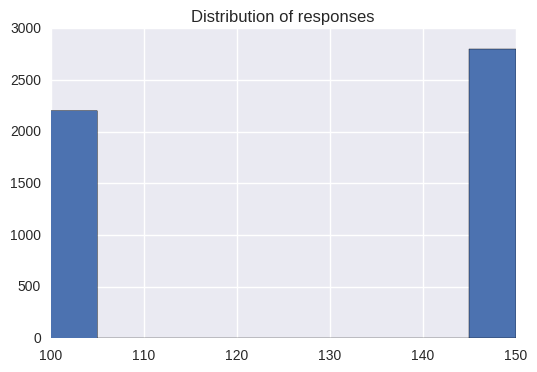### Baseline model¶ Here we quickly explore using a logistic classifier on the a basic set of features In : def try_configuration(model, features, df=df, target = 'Responder_Flg'): print('splitting dataset into training and test data...') train_ids, test_ids, xdata, xtest, ydata, ytest = split_dset(df, features, target, _seed) print('training classifier...') model.fit(xdata, ydata) print('getting model output...') train_pred = model.predict(xdata) test_pred = model.predict(xtest) train_prob = model.predict_proba(xdata)[:,1] test_prob = model.predict_proba(xtest)[:,1] train_output = pd.DataFrame({'id':train_ids, 'probability':train_prob}) test_output = pd.DataFrame({'id':test_ids, 'probability':test_prob}) # evaluate print('evaluating model...') train_cash = per_mailing_revenue(train_output) test_cash = per_mailing_revenue(test_output) print('Train per-mail-value:${0:0.2f}'.format(train_cash))
print('Test per-mail-value: ${0:0.2f}'.format(test_cash)) print('Test response rate: {0:0.4f}'.format(get_response_rate(test_output))) # ROC plot_ROC([('training_data', ydata, train_prob), ('test_data', ytest, test_prob)]) return({'train_prob': train_prob, 'test_prob':test_prob, 'train_label':ydata, 'test_label':ytest, 'model':model}) def try_logistic(features, df=df, target='Responder_Flg'): classifier = LogisticRegression(class_weight={0: 1, 1:9}, n_jobs=-1) try_configuration(classifier, features, target=target) def try_knn(features, k=5, df=df, target='Responder_Flg'): classifier = KNeighborsClassifier(5, n_jobs=-1) try_configuration(classifier, features, target=target) def try_rf(features, max_depth=None, n_estimators=10, df=df, target='Responder_Flg'): classifier = RandomForestClassifier(n_estimators=n_estimators, max_depth = max_depth, class_weight={0: 1, 1:9}, n_jobs=-1) try_configuration(classifier, features, target=target) def tree_feature_selector(features, verbose=True, df=df, target='Responder_Flg', scaling_factor = 1): print('selecting features with random forests...') train_ids, test_ids, xdata, xtest, ydata, ytest = split_dset(df, features, target, _seed) # Build a forest and compute the feature importances forest = ExtraTreesClassifier(n_estimators=250, n_jobs = -1, random_state=_seed) forest.fit(xdata, ydata) importances = forest.feature_importances_ scl = str(scaling_factor)+'*mean' model = SelectFromModel(forest, prefit=True, threshold=scl) support = model.get_support() new_feat = [] for index, include in enumerate(support): if include: new_feat.append(features[index]) if verbose: print('originally {} features'.format(len(features))) print('now {} features'.format(len(new_feat))) return new_feat  In : test_feat = [<list of five column names>] # try_logistic(test_feat) selected_feat = tree_feature_selector(test_feat) try_logistic(selected_feat) try_knn(selected_feat, df=onehot_df) try_rf(selected_feat)  selecting features with random forests... originally 5 features now 2 features splitting dataset into training and test data... training classifier... getting model output... evaluating model... Train per-mail-value:$13.92
Test per-mail-value: $13.64 Test response rate: 0.1136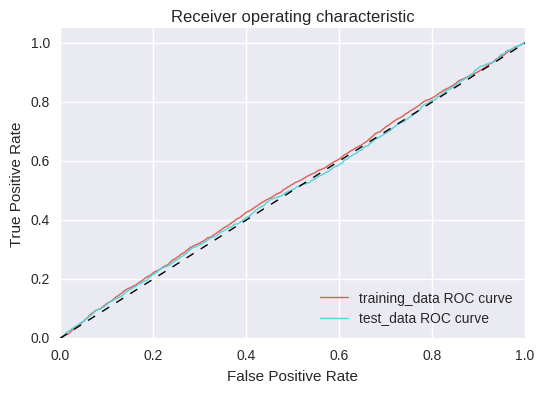splitting dataset into training and test data... training classifier... getting model output... evaluating model... Train per-mail-value:$16.31
Test per-mail-value: $14.52 Test response rate: 0.1120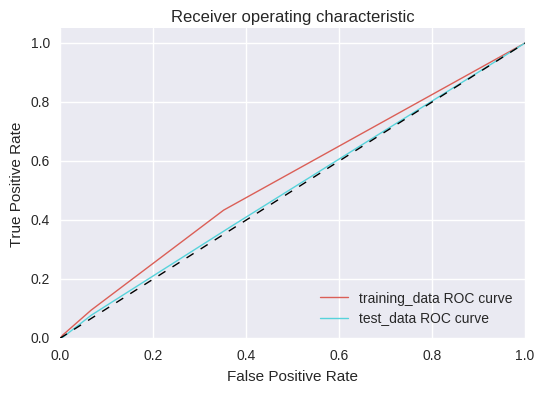splitting dataset into training and test data... training classifier... getting model output... evaluating model... Train per-mail-value:$22.69
Test per-mail-value: $15.28 Test response rate: 0.1200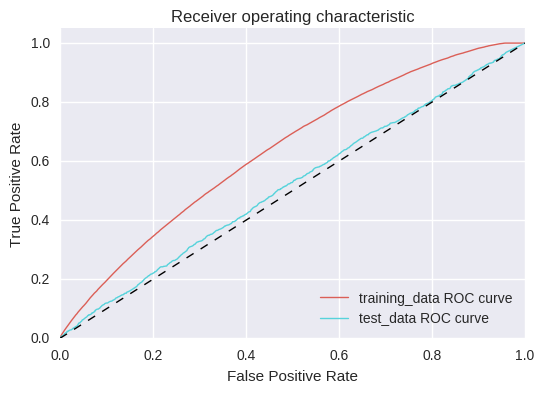In : testfeat2 = [<list of 39 column names>] selected_feat = tree_feature_selector(testfeat2) try_logistic(selected_feat) try_knn(selected_feat, 2, df=onehot_df) try_rf(selected_feat,n_estimators=100,max_depth=10)  selecting features with random forests... originally 39 features now 12 features splitting dataset into training and test data... training classifier... getting model output... evaluating model... Train per-mail-value:$16.41
Test per-mail-value: $17.12 Test response rate: 0.1376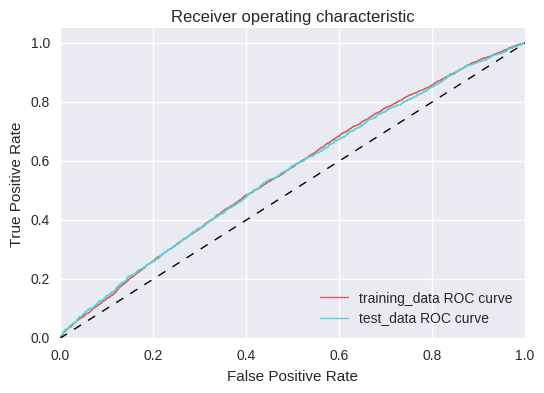splitting dataset into training and test data... training classifier... getting model output... evaluating model... Train per-mail-value:$50.68
Test per-mail-value: $13.96 Test response rate: 0.1088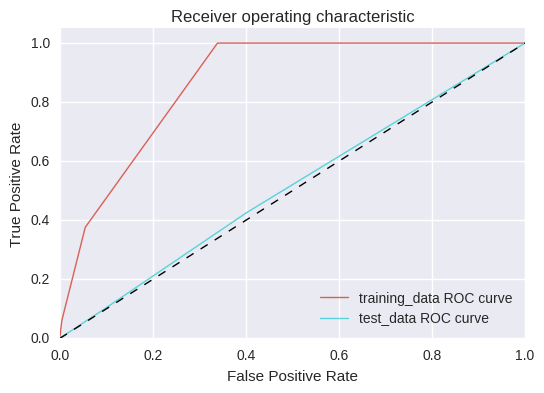splitting dataset into training and test data... training classifier... getting model output... evaluating model... Train per-mail-value:$41.19
Test per-mail-value: $18.56 Test response rate: 0.1544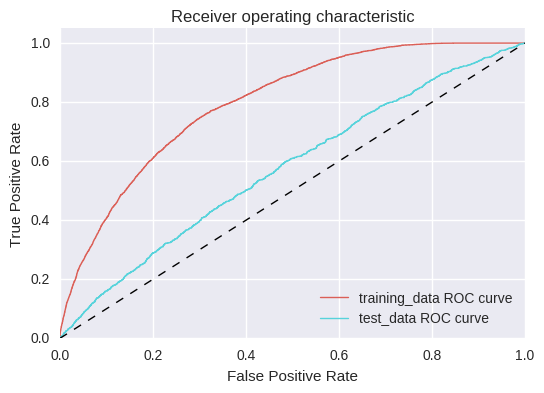In : all_feat = df.columns.values[5:] # remove ids, labels # # this can be a little slow, so we will store output as a pickle # selected_feat = tree_feature_selector(all_feat) # with open('rf_selected_features', 'wb') as fp: # pickle.dump(selected_feat, fp)  In : # expensive computation (above), so we restore this from pickle selected_feat = [] with open('rf_selected_features', 'rb') as fp: selected_feat = pickle.load(fp)  In : # try_logistic(selected_feat, df=onehot_df) # using one-hot encoding doesn't actually seem to make a big difference, here (same model performance) try_logistic(selected_feat) try_rf(selected_feat, n_estimators=500, max_depth=4)  splitting dataset into training and test data... training classifier... getting model output... evaluating model... Train per-mail-value:$18.68
Test per-mail-value: $18.20 Test response rate: 0.1464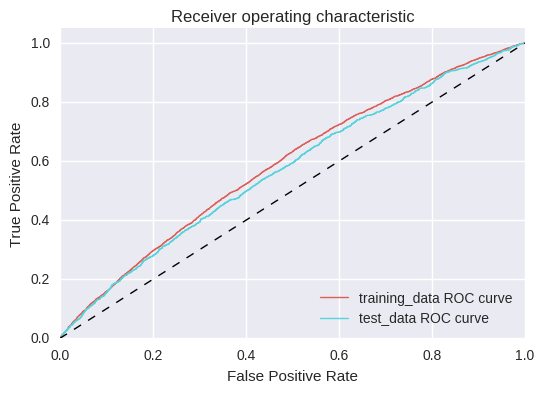splitting dataset into training and test data... training classifier... getting model output... evaluating model... Train per-mail-value:$22.57
Test per-mail-value: $17.88 Test response rate: 0.1504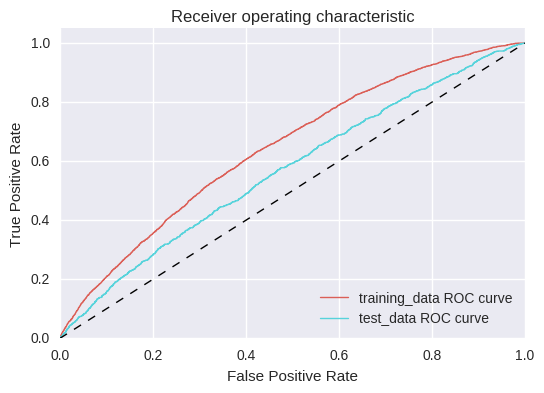## Step 3: Just throw XGBOOST at it!¶ XGBOOST is the black-box algorithm that dominates at the top tiers of data science competitions on Kaggle. Let's see how it does with some random parameters. Since boosting will choose the trees that do well, we don't need to worry about narrowing down our features list like before. In : print('splitting dataset into training and test data...') train_ids, valid_ids, test_ids, xdata, xvalid, xtest, ydata, yvalid, ytest = \ split_dset_with_valid(df, selected_feat, 'Responder_Flg', _seed) print('running xgboost') # Set our parameters for xgboost params = {} params['objective'] = 'binary:logistic' params['eval_metric'] = 'logloss' params['eta'] = 0.02 params['max_depth'] = 4 d_train = xgb.DMatrix(xdata, label=ydata) d_valid = xgb.DMatrix(xvalid, label=yvalid) watchlist = [(d_train, 'train'), (d_valid, 'valid')] bst = xgb.train(params, d_train, 400, watchlist, early_stopping_rounds=50, verbose_eval=10)  splitting dataset into training and test data... running xgboost  train-logloss:0.680505 valid-logloss:0.680377 Multiple eval metrics have been passed: 'valid-logloss' will be used for early stopping. Will train until valid-logloss hasn't improved in 50 rounds.  train-logloss:0.577662 valid-logloss:0.576527  train-logloss:0.506042 valid-logloss:0.504155  train-logloss:0.454934 valid-logloss:0.452535  train-logloss:0.417977 valid-logloss:0.415153  train-logloss:0.390992 valid-logloss:0.38794  train-logloss:0.371225 valid-logloss:0.367938  train-logloss:0.356737 valid-logloss:0.353288  train-logloss:0.346045 valid-logloss:0.342606  train-logloss:0.338135 valid-logloss:0.334806  train-logloss:0.332262 valid-logloss:0.329095  train-logloss:0.327802 valid-logloss:0.324873  train-logloss:0.324432 valid-logloss:0.321775  train-logloss:0.3218 valid-logloss:0.319486  train-logloss:0.319681 valid-logloss:0.317902  train-logloss:0.317913 valid-logloss:0.316822  train-logloss:0.31647 valid-logloss:0.315937  train-logloss:0.315178 valid-logloss:0.315315  train-logloss:0.314034 valid-logloss:0.314905  train-logloss:0.312979 valid-logloss:0.314613  train-logloss:0.312007 valid-logloss:0.314322  train-logloss:0.311087 valid-logloss:0.314239  train-logloss:0.310266 valid-logloss:0.31412  train-logloss:0.309449 valid-logloss:0.314095  train-logloss:0.308598 valid-logloss:0.314047  train-logloss:0.307761 valid-logloss:0.313994  train-logloss:0.307005 valid-logloss:0.313989  train-logloss:0.306345 valid-logloss:0.313981  train-logloss:0.305617 valid-logloss:0.313997  train-logloss:0.305 valid-logloss:0.31404  train-logloss:0.304239 valid-logloss:0.314037  train-logloss:0.303692 valid-logloss:0.313992 Stopping. Best iteration:  train-logloss:0.306418 valid-logloss:0.31397  In : d_test = xgb.DMatrix(xtest) train_prob = bst.predict(d_train) valid_prob = bst.predict(d_valid) test_prob = bst.predict(d_test) train_output = pd.DataFrame({'id':train_ids, 'probability':train_prob}) valid_output = pd.DataFrame({'id':valid_ids, 'probability':valid_prob}) test_output = pd.DataFrame({'id':test_ids, 'probability':test_prob}) # evaluate print('evaluating model...') train_cash = per_mailing_revenue(train_output) valid_cash = per_mailing_revenue(valid_output) test_cash = per_mailing_revenue(test_output) print('Train per-mail-value:${0:0.2f}'.format(train_cash))
print('Validation per-mail-value: ${0:0.2f}'.format(valid_cash)) print('Test per-mail-value:${0:0.2f}'.format(test_cash))
print('RR: {:0.4f}'.format(get_response_rate(test_output)))
plot_ROC([('training_data', ydata, train_prob), ('validation_data', yvalid, valid_prob), ('test_data', ytest, test_prob)])

evaluating model...
Train per-mail-value: $44.30 Validation per-mail-value:$17.56
Test per-mail-value: $18.88 RR: 0.1536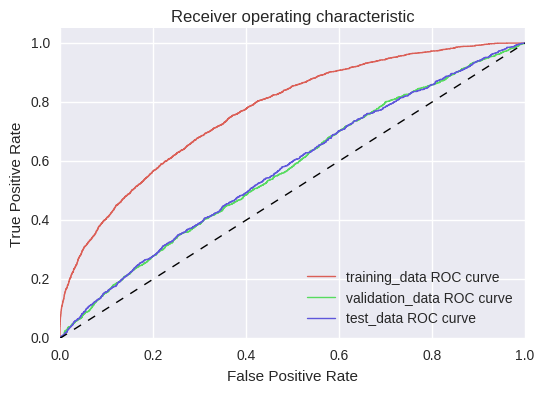# Step 4: Optimize the hyperparameters¶ We need to tune the approach to maximize our performance. We will do this via hyperparameter optimization! The gp_optimize routine approximates the function mapping hyperparameters to performance as a Gaussian Process and makes Bayesian high-likelihood informed guesses about what hyperparameters to try next. This step may actually not have done much overall, since the performance function is calculated over a very small sample of responders in the test set and is thus pretty noisy. In : # trying out skopt with scikit gb trees features = selected_feat train_ids, valid_ids, test_ids, xdata, xvalid, xtest, ydata, yvalid, ytest = \ split_dset_with_valid(df, features, 'Responder_Flg', _seed) def objective(params): # time this part start = time.time() gamma, alpha, lamb, min_child_weight, max_depth, subsample, colsample_bytree, iterations = params # Set our parameters for xgboost params = {} # these are constant params['objective'] = 'binary:logistic' params['eval_metric'] = 'logloss' params['eta'] = 0.02 # these are tuned params['gamma'] = gamma params['alpha'] = alpha params['lambda'] = lamb params['min_child_weight'] = min_child_weight params['max_depth'] = max_depth params['subsample'] = subsample params['colsample_bytree'] = colsample_bytree d_train = xgb.DMatrix(xdata, label=ydata) d_valid = xgb.DMatrix(xvalid, label=yvalid) bst = xgb.train(params, d_train, iterations) valid_prob = bst.predict(d_valid) valid_output = pd.DataFrame({'id':valid_ids, 'probability':valid_prob}) valid_cash = per_mailing_revenue(valid_output) # timing end = time.time() print('Iteration completed in {:.1f} seconds with validation return:${:.3f}'.format(end - start, valid_cash))
return -valid_cash

space  = [(0.0, 0.5),               # gamma
(0.0, 1.0),               # alpha
(0.0, 1.0),               # lambda
(1, 5),                   # min_child_weight
(2, 6),                   # max_depth
(.6, 1.0),                # subsample
(.6, 1.0),                # colsample_bytree
(200, 800),               # iterations
]

In :
from skopt import gp_minimize
# res_gp = gp_minimize(objective, space, n_calls=700, random_state=_seed)
# print('Best price achieved: ${:.3f}'.format(-res_gp.fun))  In : # # get best params # gamma, alpha, lamb, min_child_weight, max_depth, subsample, colsample_bytree, iterations = res_gp.x # # Set our parameters for xgboost # params = {} # # these are constant # params['objective'] = 'binary:logistic' # params['eval_metric'] = 'logloss' # params['eta'] = 0.02 # # these are tuned # params['gamma'] = gamma # params['alpha'] = alpha # params['lambda'] = lamb # params['min_child_weight'] = min_child_weight # params['max_depth'] = max_depth # params['subsample'] = subsample # params['colsample_bytree'] = colsample_bytree features = selected_feat train_ids, valid_ids, test_ids, xdata, xvalid, xtest, ydata, yvalid, ytest = \ split_dset_with_valid(df, features, 'Responder_Flg', _seed) with open('optimal_params', 'rb') as fp: params = pickle.load(fp) # print('optimized parameters are {} iterations and:'.format(iterations)) # print(params) d_train = xgb.DMatrix(xdata, label=ydata) d_valid = xgb.DMatrix(xvalid, label=yvalid) d_test = xgb.DMatrix(xtest) watchlist = [(d_train, 'train'), (d_valid, 'valid')] # bst = xgb.train(params, d_train, 700, watchlist, early_stopping_rounds=25, verbose_eval=10) bst = xgb.train(params, d_train, 570) # 570 was the optimal number of iterations, but was not stored in the pickle train_prob = bst.predict(d_train) valid_prob = bst.predict(d_valid) test_prob = bst.predict(d_test) train_output = pd.DataFrame({'id':train_ids, 'probability':train_prob}) valid_output = pd.DataFrame({'id':valid_ids, 'probability':valid_prob}) test_output = pd.DataFrame({'id':test_ids, 'probability':test_prob}) # evaluate print('evaluating model...') train_cash = per_mailing_revenue(train_output) valid_cash = per_mailing_revenue(valid_output) test_cash = per_mailing_revenue(test_output) print('Train per-mail-value:${0:0.2f}'.format(train_cash))
print('Validation per-mail-value: ${0:0.2f}'.format(valid_cash)) print('Test per-mail-value:${0:0.2f}'.format(test_cash))
plot_ROC([('training_data', ydata, train_prob), ('validation_data', yvalid, valid_prob), ('test_data', ytest, test_prob)])

evaluating model...
Train per-mail-value: $60.70 Validation per-mail-value:$18.52
Test per-mail-value: $20.04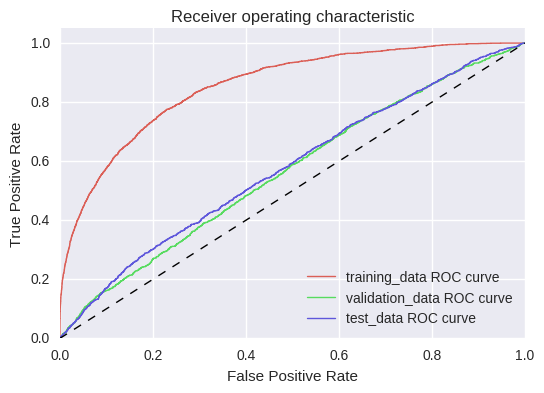In : print(get_response_rate(valid_output)) print(get_response_rate(test_output))  0.1512 0.1616  In : payment = [lookup_dict[wid] for wid in test_output['id']] test_output['payment'] = payment # test_output.sort_values(by='probability', ascending=False)[:1200]  ## Step 5: Use entire dataset to train, and make final predictions¶ We have identified a best-performing model (boosted trees with tuned hyperparameters), and so now it comes time to use the entire dataset to train the model and then to make predictions on the unlabeled dataset. With any luck this will be the winning entry! In : # prepare the datasets unlab_df = pd.read_csv('../data/testing_data.csv') unlab_df = unlab_df.set_index(df.W_ID) unlab_df.columns = [c.strip() for c in unlab_df.columns.values] # drop the column will all missing values unlab_df = unlab_df.drop('colname',1) # fill in missing values, replace missing continuous values with the average of the rows unlab_df = unlab_df.replace(-1, np.nan) cont_feats = unlab_df.select_dtypes(include=[np.number]).columns unlab_df.loc[:,cont_feats] = unlab_df.loc[:,cont_feats].fillna(unlab_df.loc[:,cont_feats].mean()) for feature in unlab_df.columns: # Loop through all columns in the dataframe if unlab_df[feature].dtype.name == 'object': # Only apply for columns with categorical strings unlab_df[feature] = pd.Categorical(unlab_df[feature]).codes # Replace strings with an integer target = 'Responder_Flg' features = [] with open('rf_selected_features', 'rb') as fp: features = pickle.load(fp) xtrain = df[features].as_matrix() ytrain = colvec_to_array(df[[target]].as_matrix()) xtest = unlab_df[features].as_matrix() train_ids = colvec_to_array(df['W_ID'].as_matrix()) test_ids = colvec_to_array(unlab_df['W_ID'].as_matrix())  In : d_train = xgb.DMatrix(xtrain, label=ytrain) d_test = xgb.DMatrix(xtest) params = {} with open('optimal_params', 'rb') as fp: params = pickle.load(fp) bst = xgb.train(params, d_train, 570) train_prob = bst.predict(d_train) test_prob = bst.predict(d_test) train_output = pd.DataFrame({'id':train_ids, 'probability':train_prob}) test_output = pd.DataFrame({'id':test_ids, 'probability':test_prob}) # evaluate print('evaluating model...') train_cash = per_mailing_revenue(train_output) print('Train per-mail-value:${0:0.2f}'.format(train_cash))
plot_ROC([('training_data', ytrain, train_prob)])
print(get_response_rate(train_output))

evaluating model...
Train per-mail-value: \$41.68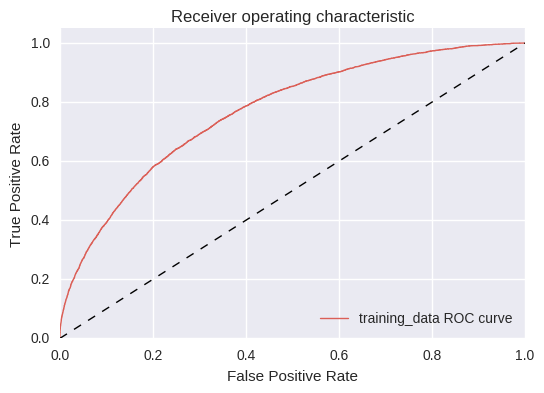0.335

In :
# results = test_output.sort_values(by='probability', ascending=False).reset_index(drop=True)
test_output.to_csv('predictions.csv', index=False)